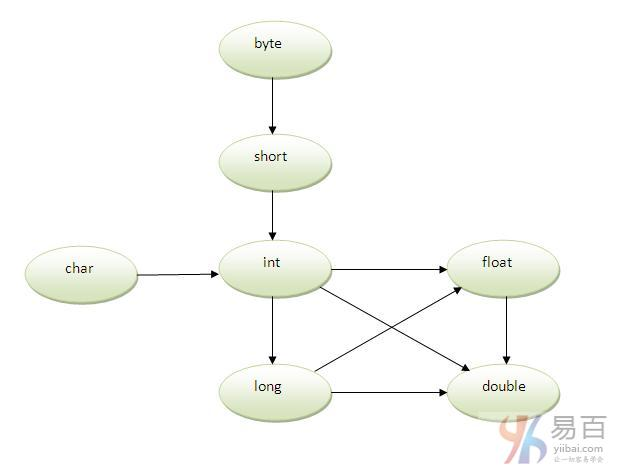# Java方法重载

## 更多精彩内容以及学习资料，尚学堂论坛bbs.bjsxt.com免费下载。

• 方法重载提高了程序的可读性。

• 通过改变参数的数量
• 通过更改数据类型

## 1. 方法重载： 修改参数的数量

` `class Adder {     static int add(int a, int b) {         return a + b;     }      static int add(int a, int b, int c) {         return a + b + c;     } }  class TestOverloading1 {     public static void main(String[] args) {         System.out.println(Adder.add(11, 11));         System.out.println(Adder.add(11, 11, 11));     } } ``
Java

` `22 33 ``
Java

## 2. 方法重载：更改参数的数据类型

` `class Adder {     static int add(int a, int b) {         return a + b;     }      static double add(double a, double b) {         return a + b;     } }  class TestOverloading2 {     public static void main(String[] args) {         System.out.println(Adder.add(11, 11));         System.out.println(Adder.add(12.3, 12.6));     } } ``
Java

` `22 24.9 ``
Java

` `class Adder {     static int add(int a, int b) {         return a + b;     }      static double add(int a, int b) {         return a + b;     } }  class TestOverloading3 {     public static void main(String[] args) {         System.out.println(Adder.add(11, 11));// ambiguity     } } ``
Java

` `Compile Time Error: method add(int,int) is already defined in class Adder ``
Java

`System.out.println(Adder.add(11,11));`//这里，java如何确定应该调用哪个`sum()`方法？

` `class TestOverloading4{       public static void main(String[] args){System.out.println("main with String[]");}       public static void main(String args){System.out.println("main with String");}       public static void main(){System.out.println("main without args");}   } ``
Java

` `main with String[] ``
Java

## 方法重载和类型提升` `class OverloadingCalculation1 {     void sum(int a, long b) {         System.out.println(a + b);     }      void sum(int a, int b, int c) {         System.out.println(a + b + c);     }      public static void main(String args[]) {         OverloadingCalculation1 obj = new OverloadingCalculation1();         obj.sum(20, 20);// now second int literal will be promoted to long         obj.sum(20, 20, 20);      } } ``
Java

` `40 60 ``
Java

` `class OverloadingCalculation2 {     void sum(int a, int b) {         System.out.println("int arg method invoked");     }      void sum(long a, long b) {         System.out.println("long arg method invoked");     }      public static void main(String args[]) {         OverloadingCalculation2 obj = new OverloadingCalculation2();         obj.sum(20, 20);// now int arg sum() method gets invoked     } } ``
Java

` `Output:int arg method invoked ``
Java

` `class OverloadingCalculation3 {     void sum(int a, long b) {         System.out.println("a method invoked");     }      void sum(long a, int b) {         System.out.println("b method invoked");     }      public static void main(String args[]) {         OverloadingCalculation3 obj = new OverloadingCalculation3();         obj.sum(20, 20);// now ambiguity     } } ``
Java

## 相关资讯

• #### 学堂故事

•尚学堂微博尚学堂订阅号尚学堂服务号

• 北京校区
• 西安校区
• 山西校区
• 武汉校区
• 深圳校区
• 上海校区
• 广州校区
• 保定招生办
• 黑龙江项目办

Copyright 2006-2021 北京尚学堂科技有限公司  京ICP备13018289号-19  京公网安备11010802015183   营业执照